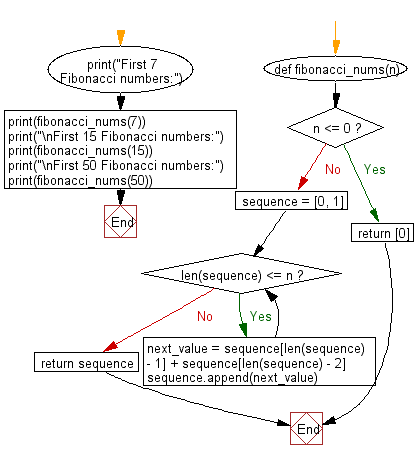﻿ Python: Generate a list, containing the Fibonacci sequence, up until the nth term - w3resource# Python: Generate a list, containing the Fibonacci sequence, up until the nth term

## Python List: Exercise - 265 with Solution

Write a Python program to generate a list, containing the Fibonacci sequence, up until the nth term.

• Starting with 0 and 1, use list.append() to add the sum of the last two numbers of the list to the end of the list, until the length of the list reaches n.
• If n is less or equal to 0, return a list containing 0.

Sample Solution:

Python Code:

``````def fibonacci_nums(n):
if n <= 0:
return 
sequence = [0, 1]
while len(sequence) <= n:
next_value = sequence[len(sequence) - 1] + sequence[len(sequence) - 2]
sequence.append(next_value)
return sequence
print("First 7 Fibonacci numbers:")
print(fibonacci_nums(7))
print("\nFirst 15 Fibonacci numbers:")
print(fibonacci_nums(15))
print("\nFirst 50 Fibonacci numbers:")
print(fibonacci_nums(50))
```
```

Sample Output:

```First 7 Fibonacci numbers:
[0, 1, 1, 2, 3, 5, 8, 13]

First 15 Fibonacci numbers:
[0, 1, 1, 2, 3, 5, 8, 13, 21, 34, 55, 89, 144, 233, 377, 610]

First 50 Fibonacci numbers:
[0, 1, 1, 2, 3, 5, 8, 13, 21, 34, 55, 89, 144, 233, 377, 610, 987, 1597, 2584, 4181, 6765, 10946, 17711, 28657, 46368, 75025, 121393, 196418, 317811, 514229, 832040, 1346269, 2178309, 3524578, 5702887, 9227465, 14930352, 24157817, 39088169, 63245986, 102334155, 165580141, 267914296, 433494437, 701408733, 1134903170, 1836311903, 2971215073, 4807526976, 7778742049, 12586269025]
```

Flowchart:## Visualize Python code execution:

The following tool visualize what the computer is doing step-by-step as it executes the said program:

Python Code Editor:

Have another way to solve this solution? Contribute your code (and comments) through Disqus.

What is the difficulty level of this exercise?

Test your Python skills with w3resource's quiz

﻿

## Python: Tips of the Day

```print(2_000_000)
```2000000